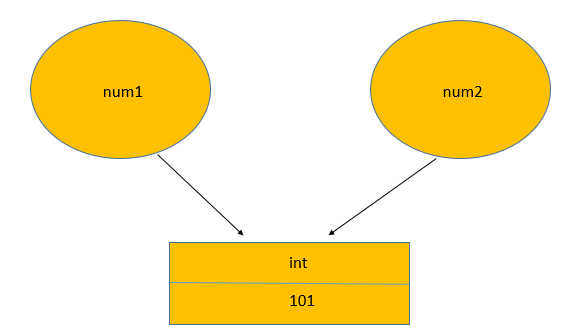Net-informations.com

# Python Identity Operators

Identity operators are used to compare the memory location of two objects, especially when both the objects have same name and can be differentiated only using its memory location. There are two Identity operators: "is" and "is not" .

1. is - Returns true if both variables are the same object.
2. is not - Returns true if both variables are not the same object.

x is y is true if and only if x and y are the same object . Object identity is determined using the id() function . x is not y yields the inverse truth value.## Is there a difference between "==" and "is"?

The is operator will return True if two variables point to the same object, "==" if the objects referred to by the variables are equal: for example in this case num1 and num2 refer to an int instance storing the value 101:Because num2 refers to the same object is and == will give True:

In the following example the names num1 and num2 refer to different int instances, even if both store the same integer:Because the same value (integer) is stored == will return True, that's why it's often called value comparison . However is operator will return False because these are different objects :

### You should only use is operator if you:

1. want to check if two objects are really the same object (not just the same "value").
1. want to compare a value to a Python constant.

#### The constants in Python are:

1. None
2. True
3. False
4. NotImplemented
5. Ellipsis
6. __debug__
7. classes (for example int is int or int is float)

net-informations.com (C) 2022    Founded by raps mk